# Author a mathematical formula question

Mathematical formula questions allow instructors to compare a student response (generally a mathematical expression) to a specified answer and (in most cases) accept response equivalence.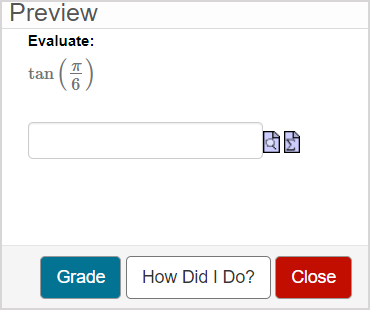There are ten sub-types of mathematical formula questions that accept specific types of responses.

TIP: Algorithmic variables can be applied to any mathematical formula question sub-type. Check out Author an algorithmic question.

TIP: All questions are authored using the Question Editor. Check out Author a question to learn how to access the Question Editor.

## To author a mathematical formula question

To author a mathematical formula question from the Question Editor:

1. Enter a question statement in the Question Text pane of the Question Editor.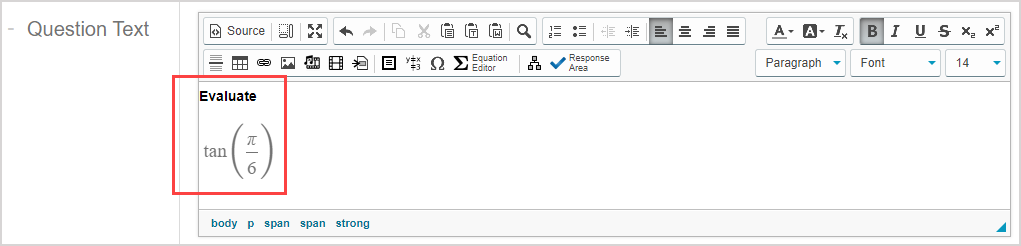TIP: Check out Use the Visual Editor for details on how to use this toolbar to the fullest to control the visual appearance of your mathematical formula question statement.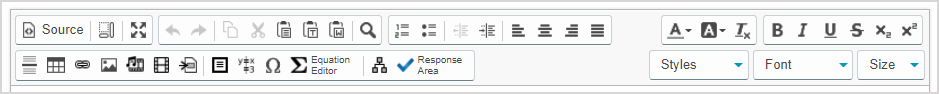1. Click Response Areato select the type of response area for this part of the question.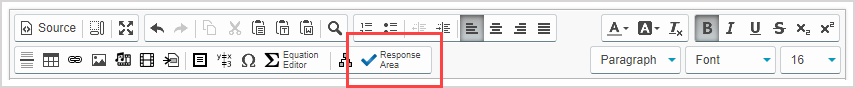NOTE: A question can have multiple question parts. Each question part have a different type of response area. Example — A question could have the first response area as a mathematical formula response area, and a second response area as multiple choice.

1. Click Mathematical Formula from the list of response area types.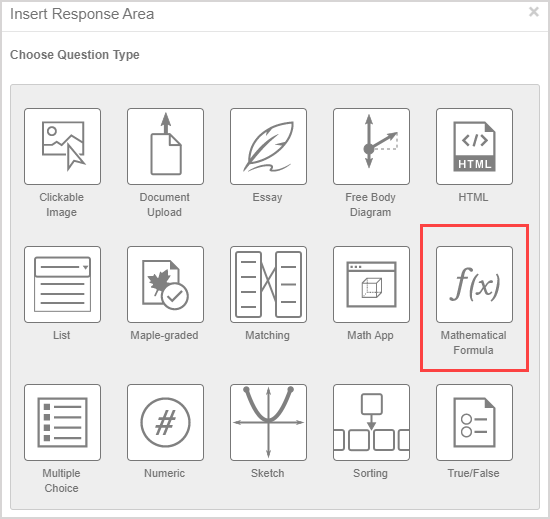1. Assign a weight to the response area. The default response area weighting is 1.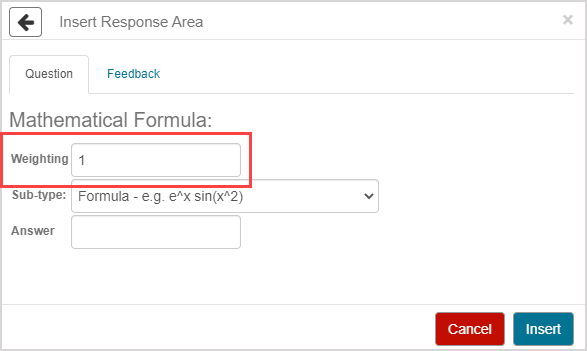TIP: The response area weight can be any integer greater than 0. Response area weighting is proportionate to the question total. Example — A question with two response areas could have the first response area weighted as 2, and the second response area weighted as 4. This means that the second response area will be worth twice as much as the first response area when calculating the total grade for the question.

1. Select the sub-type of mathematical formula question that you want to author from the Sub-type drop-down list.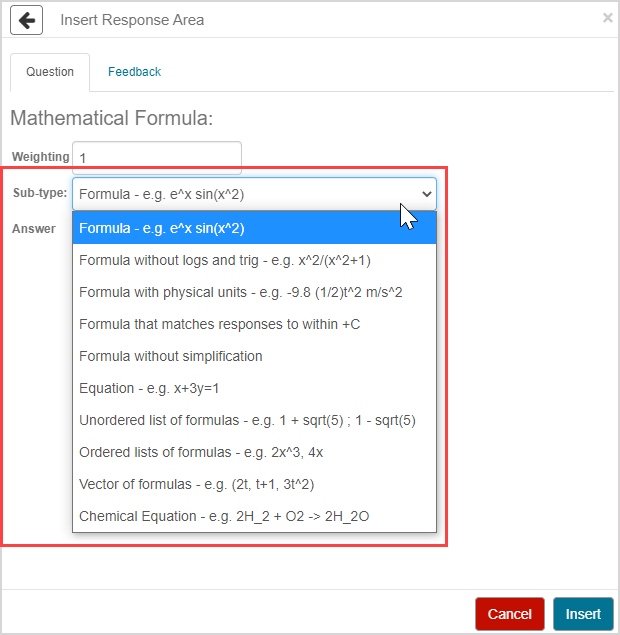1. Define the correct answer using symbolic math in the Answer field.NOTE: The answer must use the correct syntax for the selected sub-type. Examplesin can't be included in the correct answer if you're authoring a formula without logs and trig question sub-type.

1. Click Insert to add this response area to the question.1. The response area is inserted into your question.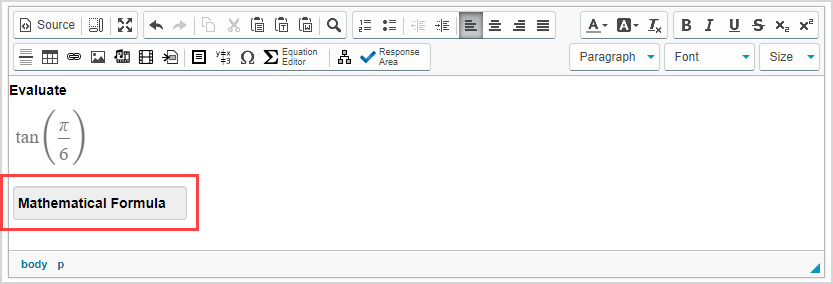1. Click Preview to trial your new question to ensure that it behaves as expected.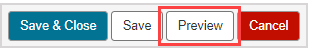TIP: You first have to click the save iconif you're creating this question from within an Activity Editor. After the question has been saved, you can then click the preview icon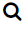to ensure that your new question behaves as expected.

1. Click Save to save your new question. Your new question is now stored in the Questions pane of the Content Repository.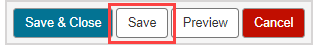TIP: Click the save iconif you're creating this question from within an Activity Editor. This saves your question to the activity and the Content Repository.

## Grading details for mathematical formula questions

Student responses are compared to the defined correct answer and must contain a mathematical expression including numbers or mathematical formulas.

In general, the ten mathematical formula question sub-types accept equivalent responses.

Here are some special notes for equivalent responses and specific sub-types:

• The formula without logs and trig sub-type won't accept logarithmic and trigonometric functions as equivalent responses.
• The formula with physical units sub-type will accept unit equivalents as long as the units are recognized by Möbius.
• The formula that matches responses to within +C sub-type accepts two answers as equivalent if they differ by a constant number.
• The formula without simplification sub-type will only accept unsimplified responses as equivalent responses.
• The equation sub-type will accept any equation that's algebraically equivalent to the correct answer.
• The chemical formula sub-type doesn't accept equivalent responses and requires the student response to be an exact match to the defined correct answer.